## Flow Control Protocols-

In computer networking, there are various flow control protocols-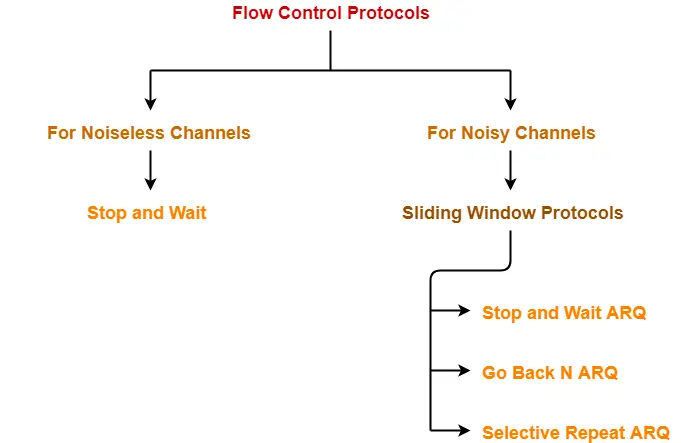## Sliding Window Protocol-

• Sliding window protocol allows the sender to send multiple frames before needing the acknowledgements.
• It is more efficient.

## Implementations-

Various implementations of sliding window protocol are-

## Comparison Table-

 Stop and Wait ARQ Go back N Selective Repeat Remarks Efficiency 1 / (1+2a) N / (1+2a) N / (1+2a) Go back N and Selective Repeat gives better efficiency than Stop and Wait ARQ. Window Size Sender Window Size = 1 Receiver Window Size = 1 Sender Window Size = N Receiver Window Size = 1 Sender Window Size = N Receiver Window Size = N Buffer requirement in Selective Repeat is very large. If the system does not have lots of memory, then it is better to choose Go back N. Minimum number of sequence numbers required 2 N+1 2 x N Selective Repeat requires large number of bits in sequence number field. Retransmissions required if a packet is lost Only the lost packet is retransmitted The entire window is retransmitted Only the lost packet is retransmitted Selective Repeat is far better than Go back N in terms of retransmissions required. Bandwidth Requirement Bandwidth requirement is Low Bandwidth requirement is high because even if a single packet is lost, entire window has to be retransmitted. Thus, if error rate is high, it wastes a lot of bandwidth. Bandwidth requirement is moderate Selective Repeat is better than Go back N in terms of bandwidth requirement. CPU usage Low Moderate High due to searching and sorting required at sender and receiver side Go back N is better than Selective Repeat in terms of CPU usage. Level of difficulty in Implementation Low Moderate Complex as it requires extra logic and sorting and searching Go back N is better than Selective Repeat in terms of implementation difficulty. Acknowledgements Uses independent acknowledgement for each packet Uses cumulative acknowledgements (but may use independent acknowledgements as well) Uses independent acknowledgement for each packet Sending cumulative acknowledgements reduces the traffic in the network but if it is lost, then the ACKs for all the corresponding packets are lost. Type of Transmission Half duplex Full duplex Full duplex Go back N and Selective Repeat are better in terms of channel usage.

## Conclusions-

• Go back N is more often used than other protocols.
• SR protocol is less used because of its complexity.
• Stop and Wait ARQ is less used because of its low efficiency.
• Depending on the context and resources availability, Go back N or Selective Repeat is employed.
• Selective Repeat and Stop and Wait ARQ are similar in terms of retransmissions.
• Go back N and Selective Repeat are similar in terms of efficiency if sender window sizes are same.
• SR protocol may be considered as a combination of advantages of Stop and Wait ARQ and Go back N.
• SR protocol is superior to other protocols but because of its complexity, it is less used.

## Note-01:

Protocols at data link layer like HDLC (Low level protocols) use Go back N.

This is because-

1. Bandwidth is high
2. CPU is very busy doing routing job
3. Error rate is low since out of order packets are not possible in wired medium

## Note-02:

Protocols at transport layer like TCP (High level protocols) use selective repeat.

## Problem-01:

If the bandwidth between the sender and receiver is sufficient, CPU and buffers are moderate, then which flow control protocol would you suggest to use?

## Solution-

The suggested protocol would be Go back N.

## Problem-02:

If the bandwidth between the sender and receiver is moderate, CPU and buffers are sufficient, then which flow control protocol would you suggest to use?

## Solution-

The suggested protocol would be Selective Repeat.

To gain better understanding about sliding window protocols comparison,

Watch this Video Lecture

Next Article- Miscellaneous Problems On Flow Control Protocols

Get more notes and other study material of Computer Networks.

Watch video lectures by visiting our YouTube channel LearnVidFun.

## Flow Control Protocols-

In computer networking, there are various flow control protocols-In this article, we will discuss practice problems based on these flow control protocols.

## Problem-01:

In what protocols is it possible for the sender to receive an acknowledgement for a packet that falls outside its current window?

1. Stop and Wait
2. Selective Repeat
3. Go back N
4. All of the above

## Solution-

• Delayed Acknowledgements fall outside the current window.
• They may occur in any of the flow control protocols and received by the sender.

Thus, correct option is (D).

## Problem-02:

On a wireless link, the probability of packet error is 0.2. A stop and wait protocol is used to transfer data across the link. The channel condition is assumed to be independent from transmission to transmission. What is the average number of transmission attempts required to transfer 100 packets?

1. 100
2. 125
3. 150
4. 200

## Solution-

### Method-01:

Given-

• Probability of packet error = 0.2
• We have to transfer 100 packets

Now,

• When we transfer 100 packets, number of packets in which error will occur = 0.2 x 100 = 20.
• Then, these 20 packets will have to be retransmitted.
• When we retransmit 20 packets, number of packets in which error will occur = 0.2 x 20 = 4.
• Then, these 4 packets will have to be retransmitted.
• When we retransmit 4 packets, number of packets in which error will occur = 0.2 x 4 = 0.8 ≅ 1.
• Then, this 1 packet will have to be retransmitted.

From here, average number of transmission attempts required = 100 + 20 + 4 + 1 = 125.

Thus, Option (B) is correct.

### REMEMBER

If there are n packets to be transmitted and p is the probability of packet error, then-

Number of transmission attempts required

= n + np + np2 + np3 + …… + ∞

= n / (1-p)

Substituting the given values, we get-

Average number of transmission attempts required = 100 / (1-0.2) = 125.

Thus, Option (B) is correct.

## Problem-03:

Compute the fraction of the bandwidth that is wasted on overhead (headers and retransmissions) for a protocol on a heavily loaded 50 Kbps satellite channel with data frames consisting of 40 bits header and 3960 data bits. Assume that the signal propagation time from the earth to the satellite is 270 msec. ACK frames never occur. NAK frames are 40 bits. The error rate for data frames is 1% and the error rate for NAK frames is negligible.

1. 1.21 %
2. 2.12 %
3. 1.99 %
4. 1.71 %

## Solution-

Consider 100 frames are being sent. Then, we have-

### Useful Data Sent-

Since each frame contains 3960 data bits, so while sending 100 frames,

Useful data sent

= 100 x 3960 bits

= 396000 bits

### Useless Data Sent / Overhead-

Now,

• The error rate for data frames is 1%, therefore out of 100 sent frames, error occurs in one frame.
• This causes the negative acknowledgement to follow which causes the retransmission.

So, we have-

• Overhead due to headers = 100 x 40 bits = 400 bits.
• Overhead due to negative acknowledgement = 40 bits.
• Overhead due to retransmission = 40 bits header + 3960 data bits = 4000 bits.

From here,

= 400 bits + 40 bits + 4000 bits

= 8040 bits

### Calculating Efficiency-

Efficiency (η) = Useful data sent / Total data sent

Here,

• Useful data sent = 396000 bits
• Total data sent = Useful data sent + Overhead = 396000 bits + 8040 bits = 404040 bits

Substituting the values, we get-

Efficiency (η)

= 396000 bits / 404040 bits

= 0.9801

### Calculating Bandwidth Utilization-

Bandwidth Utilization

= Efficiency x Bandwidth

= 0.9801 x 50 Kbps

= 49.005 Kbps

### Calculating Bandwidth Wasted-

Bandwidth wasted

= Bandwidth – Bandwidth Utilization

= 50 Kbps – 49.005 Kbps

= 0.995 Kbps

### Calculating Fraction of Bandwidth Wasted-

Fraction of bandwidth wasted

= Wasted Bandwidth / Total Available Bandwidth

= 0.995 Kbps / 50 Kbps

= 0.0199

= 1.99 %

Thus, Option (C) is correct.

## Problem-04:

Consider 1 Mbps error free line. The maximum frame size is 1000 bits. New packets are generated about 1 sec apart. The time out interval is 10 msec. If the ack timer is eliminated. How many times the average message be transmitted?

1. Only once
2. Twice
3. Thrice
4. Can’t say

## Solution-

• Transmission delay (Tt) = L / B = 1000 bits / 106 bits per sec = 1 msec.
• After packet is put on the link, the time out timer is started which is 10 msec long.
• The next packet is transmitted after 1 sec = 1000 msec.
• If no acknowledgement is received within 10 msec, the packet will be retransmitted.
• We have been asked how many times the average message be transmitted i.e. how many retransmissions are possible.
• Retransmission occurs or not depends on the propagation delay (Tp).
• If Tp is more, time out will occur and retransmission will take place but if Tp is less, then there will be no time out.
• Since propagation delay (Tp) is not given in the question, therefore we can not say anything.

Thus, Option (D) is correct.

## Problem-05:

What is the effect on line utilization if we increase the number of frames for a constant message size?

1. Lower line efficiency
2. Higher line efficiency
3. No change in line efficiency
4. No relation between line efficiency and frame size

## Solution-

In both the following cases, line utilization remains the same-

• Whether the entire message is sent as a single entity
• Or the entire message is divided into frames and then frames are sent.

This is because line contains the same amount of data in both cases.

So,

• If the number of frames are increased by dividing the message, there is no change in line efficiency.
• The line efficiency remains the same.

Thus, Option (C) is correct.

Next Article- Flow Control Methods | Single Parity Check

Get more notes and other study material of Computer Networks.

Watch video lectures by visiting our YouTube channel LearnVidFun.

## Selective Repeat Protocol-

Before you go through this article, make sure that you have gone through the previous article on Selective Repeat Protocol.

We have discussed-

• Sliding Window Protocols allow the sender to send multiple frames before needing acknowledgements.
• Selective Repeat is an implementation of a sliding window protocol.

In this article, we will discuss practice problems based on selective repeat protocol.

## Problem-01:

The maximum window size for data transmission using the selective repeat protocol with n bit frame sequence numbers is-

1. 2n
2. 2n-1
3. 2n-1
4. 2n-2

## Solution-

We know-

• With n bits, total number of sequence numbers possible = 2n.
• In SR Protocol, sender window size = receiver window size = W (say)

For any sliding window protocol to work without any problems,

 Min Available Sequence Numbers = Sender window size + Receiver window size

So, we have-

2n = W + W

2n = 2W

W = 2n-1

Therefore, maximum window size possible of sender and receiver = 2n-1

Thus, Option (B) is correct.

## Problem-02:

In SR protocol, suppose frames through 0 to 4 have been transmitted. Now, imagine that 0 times out, 5 (a new frame) is transmitted, 1 times out, 2 times out and 6 (another new frame) is transmitted.

At this point, what will be the outstanding packets in sender’s window?

1. 341526
2. 3405126
3. 0123456
4. 654321

## Solution-

In SR Protocol, only the required frame is retransmitted and not the entire window.

### Step-01:

Frames through 0 to 4 have been transmitted-

4 , 3 , 2 , 1 , 0

### Step-02:

0 times out. So, sender retransmits it-

0 , 4 , 3 , 2 , 1

### Step-03:

5 (a new frame) is transmitted-

5 , 0 , 4 , 3 , 2 , 1

### Step-04:

1 times out. So, sender retransmits it-

1 , 5 , 0 , 4 , 3 , 2

### Step-05:

2 times out. So, sender retransmits it-

2 , 1 , 5 , 0 , 4 , 3

### Step-06:

6 (another new frame) is transmitted-

6 , 2 , 1 , 5 , 0 , 4 , 3

Thus, Option (B) is correct.

## Problem-03:

The selective repeat protocol is similar to Go back N except in the following way-

1. Frame Formats are similar in both the protocols
2. The sender has a window defining maximum number of outstanding frames in both the protocols
3. Both uses piggybacked acknowledgements where possible and does not acknowledge every frame explicitly.
4. Both uses piggyback approach that acknowledges the most recently received frame

## Solution-

Also Read- Go back N Protocol

### Option (A)-

• Both the protocols use the same frame formats because both are sliding window protocols.
• The variation occurs only in the coding and implementation.

### Option (B)-

• In both the protocols, sender has a window which defines the maximum number of outstanding frames.

### Option (C)-

• Both the protocols use piggybacked acknowledgements wherever possible.
• Sending acknowledgements along with the data are called as piggybacked acknowledgements.
• But Go back N protocol uses cumulative acknowledgements and does not acknowledge every frame explicitly.
• On the other hand, Selective repeat protocol acknowledges each frame independently.

### Option (D)-

• Both the protocols use piggyback approach.
• Go back N acknowledges the most recently received frame by sending a cumulative acknowledgement which includes the acknowledgement for previous packets too if any.
• On the other hand, Selective Repeat protocol acknowledges all the frames independently and not only the recently received frame.

Thus, Options (C) and (D) are correct.

## Problem-04:

Consider a 128 x 103 bits/sec satellited communication link  with one way propagation delay of 150 msec. Selective Retransmission (repeat) protocol is used on this link to send data with a frame size of 1 KB. Neglect the transmission time of acknowledgement. The minimum number of bits required for the sequence number field to achieve 100% utilization is ________ .

## Solution-

Given-

• Bandwidth = 128 x 103 bits/sec
• Propagation delay (Tp) = 150 msec
• Frame size = 1 KB

Now,

• To achieve 100% utilization, efficiency must be 100%.
• Efficiency is 100% when sender window size is optimal i.e. 1+2a

### Calculating Transmission Delay-

Transmission delay (Tt)

= Frame size / Bandwidth

= 1 KB / (128 x 103 bits per sec)

= (1 x 210 x 8 bits) / (128 x 103 bits per sec)

= 64 msec

### Calculating Value of ‘a’-

a = Tp / Tt

a = 150 msec / 64 msec

a = 2.34

### Calculating Optimal Sender Window Size-

Optimal sender window size

= 1 + 2a

= 1 + 2 x 2.34

= ⌈5.68⌉

= 6

### Calculating Number Of Sequence Numbers Required-

In SR Protocol, sender window size and receiver window size are same.

So, sender window size = receiver window size = 6

Now,

For any sliding window protocol, minimum number of sequence numbers required

= Sender window size + Receiver window size

= 6 + 6

= 12

### Calculating Bits Required in Sequence Number Field-

To have 12 sequence numbers,

Minimum number of bits required in sequence number field

= ⌈log2(12)⌉

= 4

Thus,

• Minimum number of bits required in sequence number field = 4
• With 4 bits, number of sequence numbers possible = 16
• We use only 12 sequence numbers and rest 4 remains unused.

Next Article- Comparison Table Of Sliding Window Protocols

Get more notes and other study material of Computer Networks.

Watch video lectures by visiting our YouTube channel LearnVidFun.

## Sliding Window Protocol-

Before you go through this article, make sure that you have gone through the previous article on Sliding Window Protocol.

The two well known implementations of sliding window protocol are-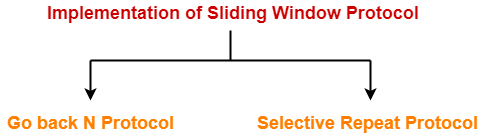1. Go back N Protocol
2. Selective Repeat Protocol

Learn about Go back N Protocol.

## Selective Repeat Protocol-

Selective Repeat protocol or SR protocol is an implementation of a sliding window protocol.

The features and working of this protocol are explained in the following points-

## Point-01:

 In SR protocol, sender window size is always same as receiver window size.

In SR protocol,

• Sender window size = Receiver window size
• The size is of course greater than 1 otherwise the protocol will become Stop and Wait ARQ.
• If n bits are available for sequence numbers, then-

Sender window size = Receiver window size = 2n/2 = 2n-1

## Point-02:

 SR protocol uses independent acknowledgements only.

In SR protocol,

• Receiver acknowledges each frame independently.
• As receiver receives a new frame from the sender, it sends its acknowledgement.

## Point-03:

 SR protocol does not accept the corrupted frames but does not silently discard them.

In SR protocol,

• If receiver receives a frame that is corrupted, then it does not silently discard that frame.
• Receiver handles the situation efficiently by sending a negative acknowledgement (NACK).
• Negative acknowledgement allows early retransmission of the corrupted frame.
• It also avoids waiting for the time out timer to expire at the sender side to retransmit the frame.

## Point-05:

 SR protocol accepts the out of order frames.

In SR protocol,

• Then, it does not discard that frame rather accepts it and keeps it in its window.

## Point-06:

 SR protocol requires sorting at the receiver’s side.

In SR protocol,

• When receiver receives a new frame, it places the new frame at the end of the linked list.
• When the received frames are out of order, receiver performs the sorting.
• Sorting sorts the frames in the correct order.

## Point-07:

 SR protocol requires searching at the sender’s side.

In SR protocol,

• Receiver does not reject the out of order frames.
• Receiver accepts the out of order frames and sort them later.
• Thus, only the missing frame has to be sent by the sender.
• For sending the missing frame, sender performs searching and finds the missing frame.
• Then, sender selectively repeats that frame.
• Thus, only the selected frame is repeated and not the entire window.
• That is why, the protocol has been named as “Selective Repeat Protocol“.

## Point-08:

 SR protocol leads to retransmission of lost frames after expiry of time out timer.

In SR protocol,

• Consider a frame being sent to the receiver is lost on the way.
• Then, it is retransmitted only after time out timer expires for that frame at sender’s side.

## Efficiency of SR Protocol-

Efficiency of any flow control protocol is given by-

 Efficiency = Sender Window Size in Protocol / (1 + 2a)

In selective repeat protocol, if sender window size = N, then-

 Efficiency of SR Protocol = N / (1 + 2a)

To gain better understanding about Selective Repeat ARQ,

Watch this Video Lecture

Next Article- Practice Problems On Selective Repeat Protocol

Get more notes and other study material of Computer Networks.

Watch video lectures by visiting our YouTube channel LearnVidFun.

## Go Back N Protocol-

Before you go through this article, make sure that you have gone through the previous article on Go back N Protocol.

We have discussed-

• Sliding window protocols allow the sender to send multiple frames before needing acknowledgements.
• Go back N is an implementation of a sliding window protocol.

In this article, we will discuss practice problems based on Go back N protocol.

## Problem-01:

A 20 Kbps satellite link has a propagation delay of 400 ms. The transmitter employs the “go back n ARQ” scheme with n set to 10.

Assuming that each frame is 100 bytes long, what is the maximum data rate possible?

1. 5 Kbps
2. 10 Kbps
3. 15 Kbps
4. 20 Kbps

## Solution-

Given-

• Bandwidth = 20 Kbps
• Propagation delay (Tp) = 400 ms
• Frame size = 100 bytes
• Go back N is used where N = 10

### Calculating Transmission Delay-

Transmission delay (Tt)

= Frame size / Bandwidth

= 100 bytes / 20 Kbps

= (100 x 8 bits) / (20 x 10bits per sec)

= 0.04 sec

= 40 msec

### Calculating Value Of ‘a’-

a = Tp / Tt

a = 400 msec / 40 msec

a = 10

### Calculating Efficiency-

Efficiency (η)

= N / (1+2a)

= 10 / (1 + 2 x 10)

= 10 / 21

= 0.476

= 47.6 %

### Calculating Maximum Data Rate Possible-

Maximum data rate possible or Throughput

= Efficiency x Bandwidth

= 0.476 x 20 Kbps

= 9.52 Kbps

≅ 10 Kbps

Thus, Correct Option is (B)

## Problem-02:

Consider the Go back N protocol with a sender’s window size of ‘n’. Suppose that at time ‘t’, the next inorder packet the receiver is expecting has a sequence number of ‘K’. Assume that the medium does not reorder messages.

### Part-01:

What are the possible sets of sequence numbers inside the sender’s window at time ‘t’. Assume the sender has already received the ACKs.

1. [K-1, K+n-1]
2. [K, K+n-1]
3. [K, K+n]
4. [K+n, K-1]

### Part-02:

If acknowledgements are still on their way to sender, what are all possible values of the ACK field in the messages currently propagating back to the sender at a time ‘t’?

1. [K-n, K-1]
2. [K-1, K-n]
3. [K, K-n]
4. [K-n, K+1]

## Solution-

### Part-01:

• In Go back N protocol, the receiver window size is 1.
• It is given that receiver expects the packet having sequence number ‘K’.
• It means it has processed all the packets ranging from 0 to K-1.
• It is given that sender has received the acknowledgement for all these packets.
• So, outstanding packets in sender’s window waiting for the acknowledgement starts from K.
• Sender window size = n.
• Therefore, last packet in sender’s window will have sequence number K+n-1.

Thus, Option (B) is correct.

### Part-02:

• Acknowledgement number is the next expected sequence number by the receiver.
• Receiver expects the packet having sequence number ‘K’ at time ‘t’.
• It means it has received the packets ranging from 0 to K-1 whose acknowledgements are are on the way.
• For the (K-1)th packet, acknowledgement number would be ‘K’.
• For the (K-2)th packet, acknowledgement number would be ‘K-1’ and so on.

Now,

• At any time, maximum number of outstanding packets can be ‘n’.
• This is because sender’s window size is ‘n’.
• Therefore, the possible values of acknowledgement number ranges from [K-n+1, ……, K-3, K-2, K-1, K] (total n values)
• Here, we have assumed that the acknowledgement for all the packets are sent independently.

Thus, Option (C) is correct.

## Problem-03:

Station A needs to send a message consisting of 9 packets to station B  using a sliding window (window size 3) and go back n error control strategy. All packets are ready and immediately available for transmission.

If every 5th packet that A transmits gets lost (but no ACKs from B ever get lost), then what is the number of packets that A will transmit for sending the message to B?

1. 12
2. 14
3. 16
4. 18

## Solution-

Given-

• Total number of packets to be sent = 9
• Go back N is used where N = 3
• Every 5th packet gets lost

### Step-01:

Since sender window size is 3, so sender sends 3 packets (1, 2, 3)-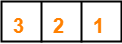Total packets sent till now from sender side = 3

### Step-02:

After receiving the acknowledgement for packet-1, sender slides its window and sends packet-4.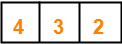Total packets sent till now from sender side = 4

### Step-03:

After receiving the acknowledgement for packet-2, sender slides its window and sends packet-5.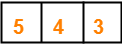Total packets sent till now from sender side = 5

### Step-04:

After receiving the acknowledgement for packet-3, sender slides its window and sends packet-6.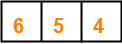Total packets sent till now from sender side = 6

### Step-05:

After receiving the acknowledgement for packet-4, sender slides its window and sends packet-7.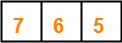Total packets sent till now from sender side = 7

### Step-06:

• According to question, every 5th packet gets lost.
• So, packet-5 gets lost and when time out occurs, sender retransmits packet-5.
• In Go back N, all the following packets are also discarded by the receiver.
• So, packet-6 and packet-7 are discarded by the receiver and they are also retransmitted.
• Thus, the entire window is retransmitted.

So, we have-Total packets sent till now from sender side = 10

Now, the next 5th packet that will be lost will be packet-7. (6, 7, 5, 6, 7)

### Step-07:

After receiving the acknowledgement for packet-5, sender slides its window and sends packet-8.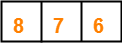Total packets sent till now from sender side = 11

### Step-08:

After receiving the acknowledgement for packet-6, sender slides its window and sends packet-9.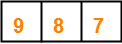Total packets sent till now from sender side = 12

### Step-09:

• According to question, every 5th packet gets lost.
• So, packet-7 gets lost and when time out occurs, sender retransmits packet-7 and the following packets.
• Thus, the entire window is retransmitted.

So, we have-Total packets sent till now from sender side = 15

Now, the next 5th packet that will be lost will be packet-9. (8, 9, 7, 8, 9)

### Step-10:

After receiving the acknowledgement for packet-7, sender slides its window.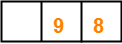Total packets sent till now from sender side = 15

### Step-11:

After receiving the acknowledgement for packet-8, sender slides its window.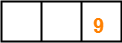Total packets sent till now from sender side = 15

### Step-12:

• According to question, every 5th packet gets lost.
• So, packet-9 gets lost and when time out occurs, sender retransmits packet-9.

So, we have-Total packets sent till now from sender side = 16

Finally, all the 9 packets got transmitted which took total 16 number of transmissions.

Thus, Correct Option is (C).

## Problem-04:

In Go back 4, if every 6th packet that is being transmitted is lost and if total number of packets to be sent is 10, then how many transmissions will be required?

## Solution-

• Try yourself!
• We have to solve in exactly the same way as we have solved Problem-03.
• Total number of transmissions required will be 17.

## Problem-05:

A 1 Mbps satellite link connects two ground stations. The altitude of the satellite is 36504 km and speed of the signal is 3 x 108 m/sec. What should be the packet size for a channel utilization of 25% for a satellite link using go back 127 sliding window protocol?

1. 120 bytes
2. 60 bytes
3. 240 bytes
4. 90 bytes

## Solution-

Given-

• Bandwidth = 1 Mbps
• Distance = 2 x 36504 km = 73008 km
• Propagation speed = 3 x 108 m/sec
• Efficiency = 25% = 1/4
• Go back N is used where N = 127

Let the packet size be L bits.

### Calculating Transmission Delay-

Transmission delay (Tt)

= Packet size / Bandwidth

= L bits / 1 Mbps

= L μsec

### Calculating Propagation Delay-

Propagation delay (Tp)

= Distance / Speed

= (73008 x 103 m) / (3 x 108 m/sec)

= 24336 x 10-5 sec

= 243360 μsec

### Calculating Value of ‘a’-

a = Tp / Tt

a = 243360 μsec / L μsec

a = 243360 / L

### Calculating Packet Size-

Efficiency (η) = N / (1+2a)

Substituting the values, we get-

1/4 = 127 / (1 + 2 x 243360 / L)

1/4 = 127 x L / (L + 486720)

L + 486720 = 508 x L

507 x L = 486720

L = 960

From here, packet size = 960 bits or 120 bytes.

Thus, Correct Option is (A).

## Problem-06:

Consider a network connecting two systems located 8000 km apart. The bandwidth of the network is 500 x 106 bits per second. The propagation speed of the media is 4 x 106 meters per second. It is needed to design a Go back N sliding window protocol for this network. The average packet size is 107 bits. The network is to be used to its full capacity.

Assume that processing delays at nodes are negligible. Then, the minimum size in bits of the sequence number field has to be ______ ?

## Solution-

Given-

• Distance = 8000 km
• Bandwidth = 500 x 106 bps
• Propagation speed = 4 x 106 m/sec
• Packet size = 107 bits

Now,

• For using the network to its full capacity, Efficiency (η) = 1
• Efficiency (η) = 1 when sender window size = 1+2a

### Calculating Transmission Delay-

Transmission delay (Tt)

= Packet size / Bandwidth

= 107 bits / (500 x 106 bits per sec)

= 1 / 50 sec

= 0.02 sec

### Calculating Propagation Delay-

Propagation delay (Tp)

= Distance / Speed

= 8000 km / (4 x 106 m/sec)

= 2 sec

### Calculating Value of ‘a’-

a = Tp / Tt

a = 2 sec / 0.02 sec

a = 100

### Calculating Sender Window Size-

Sender window size

= 1 + 2a

= 1 + 2 x 100

= 201

### Calculating Minimum Size Of Sequence Number Field-

Minimum number of bits required in the sequence number field

= ⌈log2(1+2a)⌉

= ⌈log2(201)⌉

= ⌈7.65⌉

= 8

Thus, Minimum size of sequence number field = 8 bits.

Next Article- Selective Repeat Protocol

Get more notes and other study material of Computer Networks.

Watch video lectures by visiting our YouTube channel LearnVidFun.